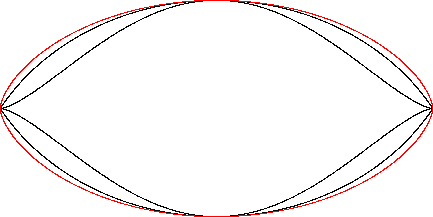Cycloid
CYCLOID

Balmoral Software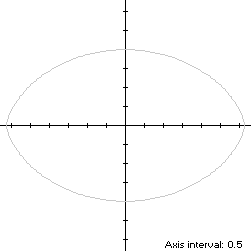Consider the closed convex shape S created from one cycloid arch, translated to the left by π and joined to its reflection across the x-axis. So that S is traversed in a counterclockwise direction around the origin starting from its right-hand corner (π,0) at t = 0, its parametric equations are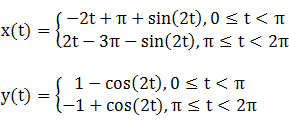Since y(π - t) = y(t) and the path of the lower arch is a point reflection through the origin of the upper arch, S is bisymmetric. Its abscissa extrema are (±π,0) and its ordinate extrema (0,±2), so the width x height of its bounding rectangle is 2π x 4. Its perimeter is double that of one cycloid arch: 2(8) = 16, and similarly, its area is 2(3π) = 6π.

### Boundary Circles & Circumellipse

The squared distance function x2(t) + y2(t) of S ranges from a minimum of 4 at (0,±2) to a maximum of π2 at (±π,0), so the inradius is 2 and the circumradius is π. Since these extrema occur on the coordinate axes, a candidate for the circumellipse is one enclosed by the annulus between the two boundary circles, with a = π and b = 2. For verification, we have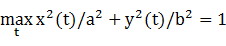### Inellipse

In Lemma B, we have for the upper arch
x(t)y(t) = [-2t + π + sin(2t)][1 - cos(2t)]
This expression is maximized at
t* = 0.984995,
so the inellipse dimensions are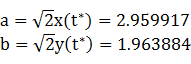For verification, we have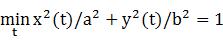### Summary Table

 Perimeter Area Centroid Figure Parameters Incircle R = 2 12.566371 12.566371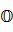Inellipse a = 2.959917b = 1.963884 15.627233 18.261870 Cycloid arches Width: 2πHeight: 4 16 18.849556 Circumellipse a = πb = 2 16.352486 19.739211 Circumcircle R = π 19.739209 31.006277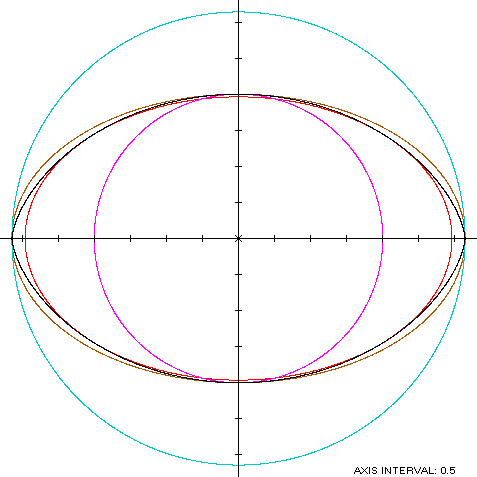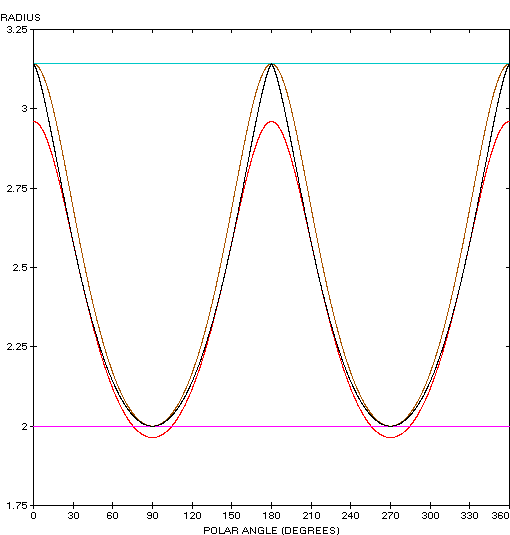The cycloid (red) is a member of a group of similarly-shaped figures described on these pages, including (inside to outside) the mouth curve and the Vesica Piscis: